# Class 10 Science: CBSE Sample Question Paper- Term I (2021-22) - 3 Notes | Study CBSE Sample Papers For Class 10 - Class 10

## Class 10: Class 10 Science: CBSE Sample Question Paper- Term I (2021-22) - 3 Notes | Study CBSE Sample Papers For Class 10 - Class 10

The document Class 10 Science: CBSE Sample Question Paper- Term I (2021-22) - 3 Notes | Study CBSE Sample Papers For Class 10 - Class 10 is a part of the Class 10 Course CBSE Sample Papers For Class 10.
All you need of Class 10 at this link: Class 10

Class-X
Time: 90 Minutes
M.M: 40

General Instructions:

1. The Question Paper contains three sections.
2. Section A has 24 questions. Attempt any 20 questions.
3. Section B has 24 questions. Attempt any 20 questions.
4. Section C has 12 questions. Attempt any 10 questions.
5. All questions carry equal marks.
6. There is no negative marking.
Section - A

Q.1: Which of these statements is correct about the pH indicators?
(a) They are salts of strong acids and strong bases
(b) They are salts of weak acids and weak bases
(c) They are either weak acids or weak base
(d) They are either strong acids or strong base

Indicators are substances which change their colour or odour when added into an acid or an alkaline solution. laboratory to test whether a compound has acidic character or basic character, indicators are used.

Q.2: Identify the pH of a solution that turns blue litmus red.
(a) 8
(b) 6
(c) 9
(d) 10

Solutions which turn blue litmus into red are acidic solutions. Their pH is below 7.

Q.3: Reaction between an acid with a base results in the formation of
(a) A Salt
(b) Neutral acid
(c) A Vitamin
(d) All of the above

Neutralization reaction is a reaction between an acid and base to form salt and water. It is an exothermic reaction.

Q.4: Which of the following is a strong base?
(a) Ammonium Hydroxide
(b) Sodium Hydroxide
(c) Magnesium Hydroxide
(d) Copper Hydroxide

Bases which completely ionize in water to produce large amount of hydroxide ions are called strong bases. Examples include NaOH, KOH, etc.

Q.5: In the given chemical reactions, Salt X → Salt Y + Gas Z

• Salt X is commonly used in bakery product.
• Salt Y is common constituent of washing powder.
• Gas Z turns lime water milky.

The X, Y and Z in the given chemical reaction is: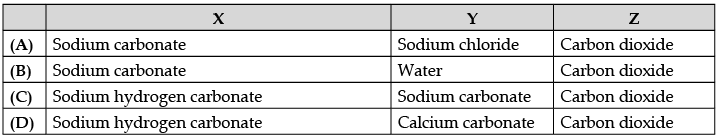In the given chemical reaction X is sodium hydrogen carbonate, Y is sodium carbonate and Z is carbon dioxide.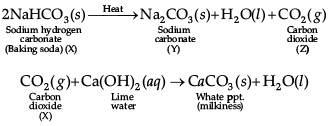Q.6: Choose the incorrect statements about metals?
(a) All metals are electropositive in nature.
(b) Metals are good reducing agents.
(c) All alkali metals can be cut by a knife.
(d) Metals form negative ions by gaining electrons.

Non-metals are elements which form negative ions by gaining electrons. Thus, they are also known as electronegative elements.

Q.7: In the given chemical reaction, which metal is more reactive?
FeSO4 + Zn → ZnSO4 + Fe
(a) Fe
(b) Zn
(c) O2
(d) All of these

Zinc is more reactive than Iron. When Zinc is added to Iron (II) Sulphate solution, Zinc displaces Iron from its solutions and Zinc sulphate is formed.
Zn(s) + FeSO4(aq) → ZnSO4(aq) + Fe(s)

Q.8: An element “Z” reacts with oxygen to form oxides of Z which turns blue litmus solution to red. The element Z is:
(a) A Metal
(b) A Non-metal
(c) Both (A) and (B)
(d) None of these

Non-metals react with oxygen to form acidic oxides or neutral oxides. These acidic oxides dissolve in water to form acids.
Non-metal + Oxygen Non-metallic oxide

Q.9: The electronic configuration of three elements is given below.
X- 2,8,2
Y- 2,8,6
Z- 2,8,1

Which two elements will combine to form an ionic compound?
(a) X and Y
(b) Y and Z
(c) X and Z
(d) Both (A) and (B)

(X and Y) and (Y and Z) will form an ionic compound because when one of the atoms donate electrons to achieve the inert gas configuration, and the other atom needs electrons to achieve the inert gas configuration, an ionic bond is formed. As X gas 2 and Y gas 6 electrons, also Y has 6 and Z has 1 electron in their outermost shell, they would form an ionic compound.

Q.10: Based on the given two reactions, find the least and the most reactive metal.
I. FeSO4 + Zn → ZnSO4 + Fe
II. CuSO4 + Fe → FeSO4 + Cu
Most reactive Least reactive
(a) Cu Zn
(b) Zn Fe
(c) Zn Cu
(d) Cu Fe

In the first reaction, Zn displaces Fe from FeSO4. So Zn is more reactive than Fe while in the second reaction, Fe displaces Cu from CuSO4. So Fe is more reactive than Cu. Hence, Zn is the most reactive metal and Cu is the least reactive metal.

Q.11: Which of these regulate the opening and closing of stomata?
(a) Lenticel
(b) Guard Cells
(c) Hydathodes
(d) All the above

The opening and closing of stomatal pores are controlled by the turgidity of guard cells. When guard cells uptake water from surrounding cells, they swell to become a turgid body. This enlarges the pore in between and causing stomatal opening. When water is released, they become flaccid. This closes the pore in between causing stomatal closing.

Q.12: Match and choose the correct options: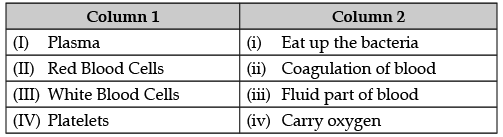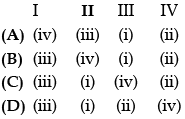Plasma is the fluid part of the blood, red blood cells carry oxygen, white blood cells eat the bacteria, and platelets helps in coagulation of blood.

Q.13. Which of the following is not present in the lymph?
(a) Protein Molecules
(b) Digested Fats
(c) Red Blood Cells

Lymph  is a yellowish fluid which escapes from the blood capillaries into the intercellular spaces. It is similar to blood but RBCs are absent in lymph.

Q.14: The heart is enclosed in a double- walled sac called:
(a) Placenta
(b) Pericardium
(c) Pleura

The heart is enclosed in a protective, double-walled sac barrier to infection and inflammation. It separate the heart from the surrounding mediastinal structures called pericardium.

Q.15. Choose the incorrect statements regarding the events of photosynthesis.
I. Light energy is absorbed by chlorophyll molecules.
II. Conversion of light energy to chemical energy and splitting of carbon dioxide into carbohydrates and oxygen.
III. Carbon dioxide is reduced into carbohydrates.
IV. Conversion of chemical energy to radiant energy and splitting of water molecules into hydrogen and oxygen.
(A) II only
(B) I and II only
(C) II and III only
(D) II and IV only

During photosynthesis, light energy is converted into chemical energy. The light absorbed by the chlorophyll results in photolysis of water i.e., splitting of water into protons (H+)  and oxygen  (O2) and evolution of electrons (e) but chemical energy does not convert into light energy in photosynthesis.

Q.16. In an experiment in order to demonstrate that a plant needs chlorophyll for photosynthesis, a student used plants that had green leaves with white areas.

After exposing the plants to sunlight, he removed a leaf from each plant and processed the leaves to remove the chlorophyll. He then tested each leaf for the presence of starch. Starch was found in the area of the leaf that was green, and no starch was found in the area of the leaf that was white.

He concluded that chlorophyll is necessary for photosynthesis.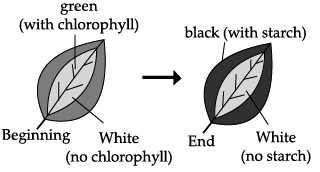Which of the given statement represents an assumption the student had to make in order to draw this conclusion?
(a) Starch is synthesized from the glucose produced in the green areas of the leaf.
(b) Starch is converted to chlorophyll in the green areas of the leaf.
(c) The white areas of the leaf do not have cells.
(d) The green areas of the leaf do not have cells.

Only green part of the leaf photosynthesis occurs and makes starch.

Q.17: Reflection is the bending of light
(a) Rays reflected back when these strike a mirror
(b) Rays reflected back when these enter a different medium
(c) Rays strike around a sharp edge
(d) Rays strike around the boundary of a narrow aperture

The phenomenon of bouncing back of light into the same medium by the smooth surface is called reflection.

Q.18: In an experiment, a ray of light was made to enter a glass slab from air. Choose the correct statement.
(a) Its wavelength will decrease.
(b) Its wavelength will increase.
(c) Its frequency will increase.
(d) None of these

When a beam of light passes from a denser medium to a rarer medium, its velocity increases and wavelength decreases, but frequency remains constant.

Q.19: The magnification of mirror is ___I___ in case of a virtual and erect image and ____II____ in case of real and inverted image.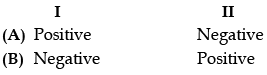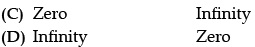Magnification of a mirror is the ratio between the height of the image hi to the height of the object ho. For a real and inverted image, the height of the image is negative and the height of the object is positive. So the magnification of the mirror is negative. If the image is erect then the image height will be taken as positive according to sign convention so, the magnification of a virtual erect image is always positive.

Q.20: ____I____ mirror is used in automobiles to see the rear view while ___II____ mirror is used in search lights.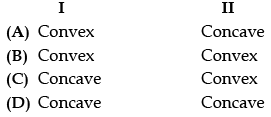The mirror used in automobiles to see the rear view is always convex. This is because convex mirror produces image that is formed at the focus, extremely diminished, virtual and erect when the object is at infinity. Concave mirrors are used in so that light beam. It is used for different light rays a single point.

Q.21: An object is kept within the focus of a concave mirror, an enlarged image is formed behind the mirror.
(a) Real
(b) Inverted
(c) Virtual and Inverted
(d) Virtual and Erect

An object is kept within the focus of a concave mirror, an enlarged image is formed behind the mirror.

Q.22: Which of these statements is correct about focal length?
(a) It is the distance from the light source to the image.
(b) It is the distance from the object to the centre of the lens.
(c) It is the distance from the image to the centre of the lens.
(d) It is the distance from the focal point to the centre of the lens.

Focal length is the distance from the focal point to the centre of the lens.

Q.23: Choose the correct statements.
I. The principal focus of a converging lens is real.

II. The principal focus of a diverging lens is virtual.

III. The principal focus of a lens always lies on the principal axis.
(a) I and II only
(b) II and III only
(c) I and III only
(d) All of these

All the given three statements are correct.

Q.24: Read the following statements carefully
(i) The prism behaves same as that of rectangular glass slab.
(ii) All the colours have different angles of deviation in case of dispersion through prism
(iii) All the colours travel with the same speed of light in glass.
(iv) Dispersion of light is observed in case of rectangular glass slab.
Which of the above statement/s is true?
(a) Only (ii)
(b) (i) and (ii) only
(c) (i), (ii) and (iv) only
(d) All of the above

A prism is made up of glass in which the faces are joined at a certain angle but in case of rectangular glass slab, the opposite faces are parallel to each other. As the light enters in the prism, all the colours travel with the different speed of light because glass is a denser medium as compared to air and dispersion  of light is not observed in case of a rectangular glass slab. So, both prism and rectangular glass slab behave differently.

Section - B

Q.25: Identify the correct option from the given table which represents the type of reactions occurring in step 1 and step 2.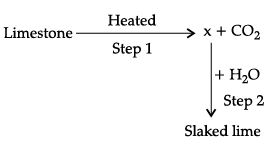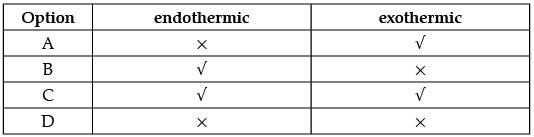Q.26: In which year is concentration of hydrogen ion the highest?
(a) 2002
(b) 2008
(c) 2011
(d) 2005

Q.27: The diagram shows the reaction between metal and dil. acid.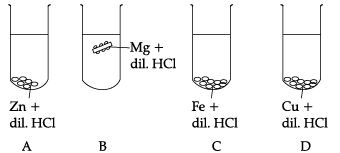What is the reason for different behaviour of Mg in test tube B?

(a) Mg is lighter element than dil. HCl
(b) Mg reacts with dil. HCl to produce H2 gas which helps in floating
(c) Mg reacts with dil. HCl to produce N2 gas which helps in floating
(d) Mg reacts with dil. HCl to produce CO2 gas which helps in floating

Q.28: The table shown below gives information about four substances: A, B, C and D.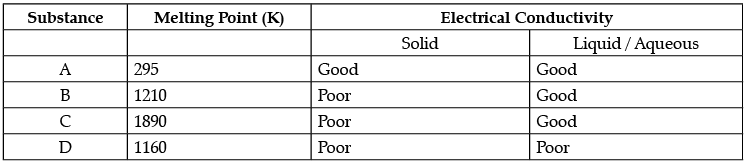Identify Ionic compounds from the above given substances.

(a) A, B
(b) B, C
(c) A, B, D
(d) A, C, D

Q.29: Vinay observed that the stain of curry on a white shirt becomes reddish-brown when soap is scrubbed on it, but it turns yellow again when the shirt is washed with plenty of water.
What might be the reason for his observation?
(i) Soap is acidic in nature
(ii) Soap is basic in nature
(iii) Turmeric is a natural indicator which gives reddish tinge in bases
(iv) Turmeric is a natural indicator which gives reddish tinge in acids
(a) i and ii
(b) ii and iii
(c) i and iv
(d) ii and iv

Q.30: In which of the following setups would the bulb glow?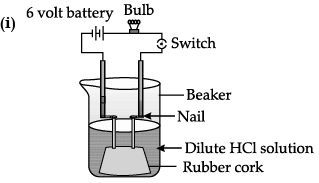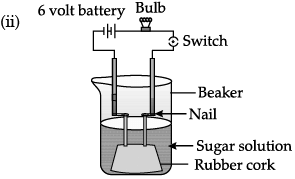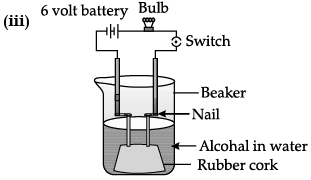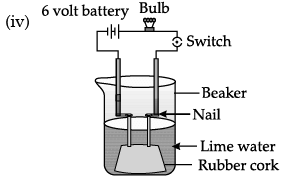(a) i and ii
(b) i and iv
(c) ii, iii and iv
(d) i, ii and iv

Q.31 to 34 consist of two statements – Assertion (A) and Reason (R). Answer these questions selecting the appropriate option given below:

Q.31: Assertion: Fresh milk in which baking soda is added, takes a longer time to set as curd.
Reason: Baking soda decreases the pH value of fresh milk to below 6.
(a) Both A and R are true and R is the correct explanation of A
(b) Both A and R are true and R is not the correct explanation of A
(c) A is true but R is false
(d) A is False but R is true

Q.32: Assertion: Decomposition of vegetable matter into compost is an endothermic reaction.
Reason: Decomposition reaction involves breakdown of a single reactant into simpler products.
(a) Both A and R are true and R is the correct explanation of A
(b)
Both A and R are true and R is not the correct explanation of A
(c)
A is true but R is false
(d)
A is False but R is true

Q.33: Assertion: Resins and gums are stored in old xylem tissue in plants.
Reason: Resins and gums facilitate transport of water molecules.
(a) Both A and R are true and R is the correct explanation of A
(b)
Both A and R are true and R is not the correct explanation of A
(c)
A is true but R is false
(d)
A is False but R is true

Q.34: Assertion: Sky appears blue in the day time.
Reason: White light is composed of seven colours
(a) Both A and R are true and R is the correct explanation of A
(b)
Both A and R are true and R is not the correct explanation of A
(c)
A is true but R is false
(d)
A is False but R is true

Q.35: The table given below shows the reaction of a few elements with acids and bases to evolve Hydrogen gas.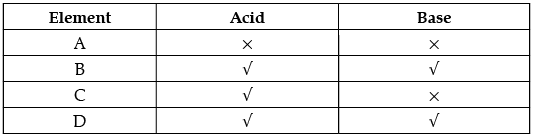Which of these elements form amphoteric oxides?
(a) A and D
(b) B and D
(c) A and C
(d) B and D

Q.36: In which of the following groups of organisms, blood flows through the heart only once during one cycle of passage through the body?

(a) Rabbit, Parrot, Turtle

(b) Frog, crocodile, Pigeon

(c) Whale, Labeo, Penguin

(d) Shark, dog fish, sting ray

Q.37: What is common between extensive network of blood vessels around walls of alveoli and in glomerulus of nephron?
(a) Thick walled arteries richly supplied with blood
(b) Thin walled veins poorly supplied with blood
(c) Thick walled capillaries poorly supplied with blood.
(d) Thin walled capillaries richly supplied with blood

Q.38: Plants use completely different process for excretion as compared to animals. Which one of the following processes is NOT followed by plants for excretion?
(a) They can get rid of excess water by transpiration.
(b) They selectively filter toxic substances through their leaves.
(c) Waste products are stored as resins and gums in old xylem.
(d) They excrete waste substances into the soil around them.

Q.39: If the power of a lens is - 4.0 D, then it means that the lens is a
(a) concave lens of focal length -50 m
(b) convex lens of focal length +50 cm
(c) concave lens of focal length -25 cm
(d) convex lens of focal length -25 m

concave lens of focal length –25 cm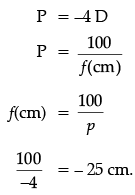Negative focal length means concave lens. Concave lens of focal length –25 cm.

Q.40: Rays from Sun converge at a point 15 cm in front of a concave mirror. Where should an object be placed so that size of its image is equal to the size of the object?
(a) 30 cm in front of the mirror
(b) 15 cm in front of the mirror
(c) Between 15 cm and 30 cm in front of the mirror
(d) More than 30 cm in front of the mirror

30 cm in front of the mirror If rays converge at a point 15 cm from the mirror, then,
f = –15 cm
then, C = – 30 cm
An object kept at C makes an image of the same size as object correct answer – (A) 30 cm in front of mirror.

Q.41: In which of the following groups of organisms, food material is broken down outside the body and then absorbed in?
(a)
mushroom, green plants, Amoeba
(b)
(c)
Paramecium, Amoeba, Cuscuta
(d)
Cuscuta, lice, tapeworm

Q.42: In a person the tubule part of the nephron is not functioning at all. What will its effect be on urine formation?
(a)
The urine will not be formed.
(b)
Quality and quantity of urine is unaffected.
(c)
Urine is more concentrated.
(d)
Urine is more diluted.

Q.43: If the real image of a candle flame formed by a lens is three times the size of the flame and the distance between lens and image is 80 cm, at what distance should the candle be placed from the lens?
(a) – 80 cm
(b) – 40 cm
(c) – 40/3 cm
(d) – 80/3 cm

m = –3
V = 80 cm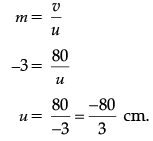Q.44: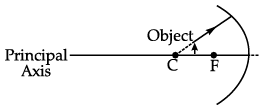While looking at the above diagram, Nalini concluded the following -
i. the image of the object will be a virtual one.
ii. the reflected ray will travel along the same path as the incident ray but in opposite direction.
iii. the image of the object will be inverted.
iv. this is a concave mirror and hence the focal length will be negative.
Which one of the above statements are correct?

(a) i and ii
(b)
i and iii
(c)
ii, iii and iv
(d)
i, ii, iii and iv

Q.45: In the above diagram light is travelling through different media. It is noted by a scientist that ∠1= ∠3= ∠4 but ∠2 <∠1. Which of the following statement would be correct?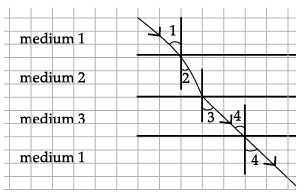(a) Medium 1 is the denser than medium 3 but it’s density is equal to medium 2.
(b) Medium 2 is the rarest medium.
(c) Medium 3 is denser than medium 1.
(d) Medium 1 and 3 are essentially the same medium, but medium 2 is denser than 1 and 3.

Q.46: The refractive index of flint glass is 1.65 and that for alcohol is 1.36 with respect to air. What is the refractive index of the flint glass with respect to alcohol ?
(a)
0.82
(b) 1.21
(c) 1.11
(d) 1.01

Refractive index of flint glass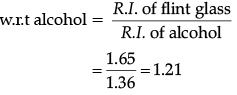Q.47: The above lens has a focal length of 10 cm. The object of height 2 mm is placed at a distance of 5 cm from the pole. Find the height of the image.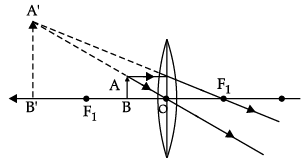(a) 4 cm
(b) 6.67 mm
(c) 4 mm
(d) 3.33 mm

f = +10 cm (Convex lens)
h1 = 2 mm = 0.2 cm.
u = – 5 cm.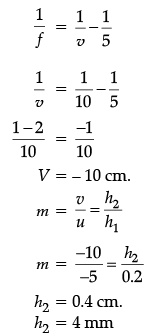Q.48: A cable manufacturing unit tested few elements on the basis of their physical properties.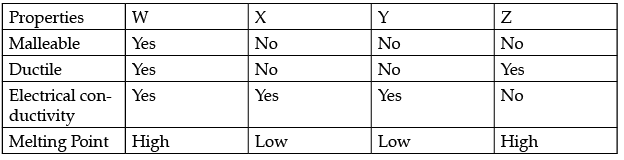Which of the above elements were dicarded for usage by the company?

(a) W, X, Y
(b)
X, Y, Z
(c)
W, X, Z
(d)
W, X, Z

Section - C

CASE-I
Observe the given experimental set-up carefully and answer the following questions from 49 to 52.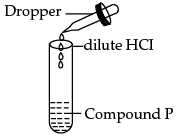Q.49: Which of the following could be the possible observation?
(a) The solution becomes blue-green due to formation of copper oxide.
(b) The solution becomes blue-green due to formation of copper chloride.
(c) The solution becomes white due to formation of calcium hydroxide.
(d) The solution becomes white due to formation of calcium oxide.

Q.50: The balanced chemical equation of the reaction which take place is:
(a) Cu(OH)2(s) + 2HCl(aq) → CuCl2(aq) + H2O(l)
(b) Cu(OH)2(s) + 2HCl(aq) → CuO(aq) + H2O(l)
(c) Cu(OH)2(s)+ HCl(aq) → CuCl2(aq) + H2O(l)
(d) CuO(s) + HCl(aq) → CuCl(aq) + H2O(l)

Q.51: On the basis of the above reaction, what can you say about the nature of compound P?
(a) It is acidic in nature
(b) It is basic in nature.
(c) It is neutral
(d) It amphoteric metal

Q.52: What you can infer from the given chemical reaction:
Hydrochloric acid + Sodium hydroxide → Sodium chloride + Water
(a)
It is a neutralization reaction
(b) It is a combustion reaction
(c) It is an oxidation reaction
(d) None of these

CASE II
The given graph shows the changes in the volume of the lungs of a person at rest over a period of 20 seconds. Study the graph and answer any of the four questions from 53 to 56.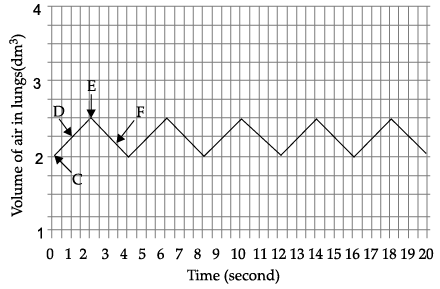Q.53: How many breaths per minute is the person taking when at rest?
(a)
5

(b) 10

(c) 15

(d) 20

Q.54: Which two points in the graph shows inspiration and expiration?
(a) D, E
(b) D, F
(c) C, D
(d) E, F

Q.55: What causes the change in air pressure during period D ?
(a) Contraction of the diaphragm muscles
(b) Decrease in the volume of the lungs
(c) Movement of ribs downward and inward
(d) Relaxation of the external intercostal muscles

Q.56: When the structure P contracts and flattens,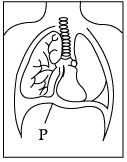(a) Air is forced out of the lungs.
(b) The volume of the thoracic cavity increases.
(c) The rib cage moves downwards and inwards.
(d) The air pressure in the lungs increases.

CASE III

Refractive index, (also called index of refraction), is the measure of the bending of a ray of light when passing from one medium into another. If i is the angle of incidence of a ray in vacuum (which is the angle between the incoming ray and the perpendicular to the surface of a medium, called the normal) and r is the angle of refraction, (which is angle between the ray in the medium and the normal), the refractive index n is defined as the ratio of the sine of the angle of incidence to the sine of the angle of refraction; i.e., n = sin i / sin r. Refractive index is also equal to the velocity of light c of a given wavelength in empty space divided by its velocity v in a substance, or n = c/v. In the given diagram, a ray of light as it travels from air to two different media A and B for a given angle of incidence can be seen.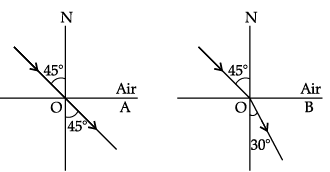Q.57: What will happen to the angle of refraction, if angle of incidence is increased in both media?
(a)
It will increase
(b) It will decrease
(c) It will remain same
(d) It will become zero in medium A.

Q.58: If n A and nB are the refractive index of two media, which of these options is correct?
(a) nA = nB
(b) nA > nB
(c) nA < nB
(d) Cannot be said.

Refractive index of medium = sin i/ sin r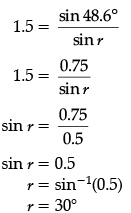Q.59: Choose the correct statement.
I. The speed of light is less in a rarer medium than a denser medium.
II. The absolute refractive index of a medium is simply called its refractive index.
III. A medium with larger refractive index is optically denser than a medium with smaller refractive index.
IV. Refraction is due to the change in speed of light as it enters from one medium to another.

(a) I and II only
(b) I, II, and III only.
(c) II, III and IV only
(d) All of these

Q.60: In an experiment, a beam of light is passed from a denser medium to a rarer medium. Based on the observation obtained, choose the correct statement.
I. Its velocity will increase
II. Its frequency remain constant
III. Its wavelength will decrease
(a)
Only I
(b)
Only II and III
(c)
Only I, and III
(d)
All of these

The document Class 10 Science: CBSE Sample Question Paper- Term I (2021-22) - 3 Notes | Study CBSE Sample Papers For Class 10 - Class 10 is a part of the Class 10 Course CBSE Sample Papers For Class 10.
All you need of Class 10 at this link: Class 10Use Code STAYHOME200 and get INR 200 additional OFF

## CBSE Sample Papers For Class 10

174 docs|7 tests

Track your progress, build streaks, highlight & save important lessons and more!

,

,

,

,

,

,

,

,

,

,

,

,

,

,

,

,

,

,

,

,

,

;